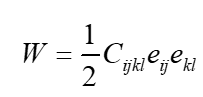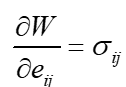# The Strain Energy Function

Trying2Learn
Summary
Where does it come from?
Earlier today, I posted a question about the strain energy function.

I am happy with the answer (I love this group).

But the answer opened up a deeper question.

Many elasticity textbook posit the existence of a strain energy function:And they make an additional assumption about its propertiesWhere does this come from? Who formulated it? I have always accepted it, but now I am questioning it.

Indeed, these two assumptions lead me down the path (along with further assumptions about the symmetries of the stress and strain tensors and related issues, and material property symmetries) to Young's Modulus and Poisson's Ratio. Thus, as statements, the "jive" with mechanical engineering results.

Are the assumptions above a case of post hoc ergo propter hoc?

Or is there any validity or justification of such assumptions, other than that they work? Is there any rational justification for such an assumption (independent of where it leads us)?

## Answers and Replies

Gold Member
Hyperelasticity or Green elasticity (which includes linear elasticity) was first developed by the mathematician George Green in the 19th century. Cauchy elasticity and hypoelasticity are other types of elasticity. All of these are general formulations that can handle nonlinear deformations.

There is also inelastic deformation which includes things like plasticity and fracture.

I am not an expert, but I believe that the primary physical assumptions of hyperelasticity are that the current state of stress depends on the current stress of strain and that the work done under loading is independent of path.

Last edited:
Trying2Learn
I am not an expert, but I believe that the primary physical assumptions of hyperelasticity are that the current state of stress depends on the current stress of strain and that the work done under loading is independent of path.

Is there any chance you can elaborate on this sentence? And show me how that formulation of strain energy is a result of assuming path independence. You have hit a nerve in me.

Gold Member
Is there any chance you can elaborate on this sentence? And show me how that formulation of strain energy is a result of assuming path independence. You have hit a nerve in me.
Sorry, but I am only able to elaborate on not being an expert.

You will probably need to look in the continuum mechanics literature which uses deformation gradients instead of strains. I believe that the strain energy density is related to the Helmholtz free energy so thermodynamics is also involved. Truesdell and Toupin/Truesdell and Noll probably have it at a very advanced level. Hopefully you can find something simpler. I believe that you will find that it is a mathematically consistent theory.

Maybe @Chestermiller or @FEAnalyst or @Andy Resnick can point you in the right direction.

Mentor
Sorry, but I am only able to elaborate on not being an expert.

You will probably need to look in the continuum mechanics literature which uses deformation gradients instead of strains. I believe that the strain energy density is related to the Helmholtz free energy so thermodynamics is also involved. Truesdell and Toupin/Truesdell and Noll probably have it at a very advanced level. Hopefully you can find something simpler. I believe that you will find that it is a mathematically consistent theory.

Maybe @Chestermiller or @FEAnalyst or @Andy Resnick can point you in the right direction.
Check out this article on the Thermodynamics of Linear Elasticity: http://maeresearch.ucsd.edu/~vlubarda/research/pdfpapers/ijss-04.pdf
I found it very powerful and very informative.

Mentor
The article I cited in post #5 is based on some very familiar thermodynamic concepts, extended to the case of a linear elastic isotropic solid:

1. The equation for the differential change in internal energy between two closely neighboring thermodynamic equilibrium states. For the case of a gas or liquid, we have $$du=Tds-Pdv$$For a linear elastic isotropic solid, the state of stress is not necessarily isotropic, and the corresponding equation for du becomes: $$du=Tds+\sigma_{ij}d\epsilon_{iij}$$where ##\sigma_{ij}## represents a component of the cartesian stress tensor, ##\epsilon_{ij}## represents a corresponding component of the cartesian small strain tensor, and where the repeated indices imply use of the Einstein summation convention.

2. The equation of state for the material. For an ideal gas, the equation of state is Pv=RT, where v is the molar volume. For an isotropic elastic solid, the corresponding equation of state at constant temperature reduces to Hooke's Law: $$\sigma_{ij}=K\epsilon_{kk}\delta_{ij}+2\mu\left[\epsilon_{ij}-\frac{\epsilon_{kk}}{3}\delta_{ij}\right]$$where, in terms of Young's modulus E and Poisson's ratio ##\nu##, the bulk modulus K and the Lame' shear modulus ##\mu## are given by $$K=\frac{E}{3(1-2\nu)}$$and$$\mu=G=\frac{E}{2(1+\nu)}$$For more general situations in which the temperature can changes so that thermal expansion can also occur, the equations of state for a linear elastic isotropic solid becomes: $$\sigma_{ij}=K(\epsilon_{kk}-\alpha(T-T_0))\delta_{ij}+2\mu\left[\epsilon_{ij}-\frac{\epsilon_{kk}}{3}\delta_{ij}\right]\tag 1$$where ##\alpha## is the coefficient of volumetric thermal expansion (3 times the coefficient of linear expansion), and where ##T_0## is the temperature of the reference configuration of the solid such that, if all the strains are zero and ##T=T_0##, the components of the stress tensor are all zero.

Eqn. 1 is obtained by expressing the total strain tensor ##\epsilon_{ij}## as the linear superposition of two successive strains: $$\epsilon_{ij}=\epsilon_{ij}^{(1)}+\epsilon_{ij}^{(2)}$$with $$\epsilon_{ij}^{(1)}=\frac{\alpha (T-T_0)}{3}\delta_{ij}$$and $$\epsilon_{ij}^{(2)}=\epsilon_{ij}-\left[\frac{\alpha (T-T_0)}{3}\right]\delta_{ij}$$The strain tensor ##\epsilon_{ij}^{(1)}## corresponds to unconstrained thermal expansion of the material by heating from temperature ##T_0## to temperature ##T##, and, because the expansion is unconstrained, results in no development of stress(##\sigma_{ij}^{(1)}=0##). The strain tensor ##\epsilon_{ij}^{(2)}## corresponds to isothermal deformation of the material with the indicated strain components.

Last edited:
Gold Member
Thinking about it, this is essentially scalar potential theory which is also used for gravity, electric fields, etc... The issue is that it is harder to determine the specific energy function and the range of it‘s applicability for materials.

FEAnalyst
@Trying2Learn I’ve found some derivation leading to the formula that you are asking about in a book titled "Podstawy mechaniki ośrodków ciągłych" (Fundamentals of continuum mechanics) by J. Ostrowska-Maciejewska. Unfortunately, it’s in Polish. It’s a bit too long to rewrite the whole derivation but if you are interested, I can send you the pictures and help with translation of descriptions.

Trying2Learn
I am probably fine as it is now. THanks anyway